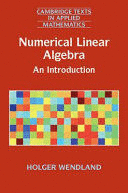# NUMERICAL LINEAR ALGEBRA. AN INTRODUCTION

Editorial:
CAMBRIDGE
Año de edición:
ISBN:
978-1-316-60117-4
Páginas:
418
Colección:
< Genérica >
\$ 954.29
IVA incluido
Sobre pedido

This self-contained introduction to numerical linear algebra provides a comprehensive, yet concise, overview of the subject. It includes standard material such as direct methods for solving linear systems and least-squares problems, error, stability and conditioning, basic iterative methods and the calculation of eigenvalues. Later chapters cover more advanced material, such as Krylov subspace methods, multigrid methods, domain decomposition methods, multipole expansions, hierarchical matrices and compressed sensing. The book provides rigorous mathematical proofs throughout, and gives algorithms in general-purpose language-independent form. Requiring only a solid knowledge in linear algebra and basic analysis, this book will be useful for applied mathematicians, engineers, computer scientists, and all those interested in efficiently solving linear problems.